Courses

# RD Sharma Solutions - Ex-11.4, Percentage, Class 7, Math Class 7 Notes | EduRev

## RD Sharma Solutions for Class 7 Mathematics

Created by: Abhishek Kapoor

## Class 7 : RD Sharma Solutions - Ex-11.4, Percentage, Class 7, Math Class 7 Notes | EduRev

The document RD Sharma Solutions - Ex-11.4, Percentage, Class 7, Math Class 7 Notes | EduRev is a part of the Class 7 Course RD Sharma Solutions for Class 7 Mathematics.
All you need of Class 7 at this link: Class 7

#### Question 1:

Find each of the following:
(i) 7% of Rs 7150
(ii) 40% of 400 kg
(iii) 20% of 15.125 litres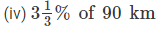(v) 2.5% of 600 metres

(i)  7% of  Rs. 7150 = (7/100) × 7150 = 50050/100 = Rs.  500.50
(ii) 40% of 400 kg = (40/100) ×  400 = 16000/100 = 160 kg
(iii) 20% of 15.125 litres = (20/100) ×  15.125 =  302.5/100 = 3.025 litres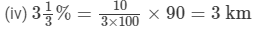(v) 2.5% of 600 metres = (2.5/100) × 600 = 1500/100 = 15 metres

#### Question 2:

Find the number whose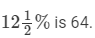Let the number be x.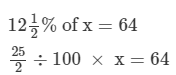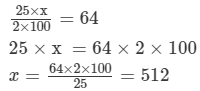#### Question 3:

What is the number,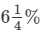of which is 2?

Let the number be x.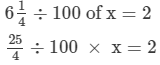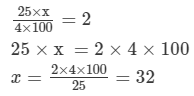#### Question 4:

If 6 is 50% of a number, what is that number?

Let the number be x.
50% of x = 6
⇒ (50/100) × x = 6
⇒ x = 600/50
⇒ x = 12
So, the number is 12.

97 docs

,

,

,

,

,

,

,

,

,

,

,

,

,

,

,

,

,

,

,

,

,

,

,

,

,

,

,

,

,

,

;